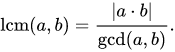# Mathematical concepts

Tell us what’s happening:
ignore my code, iv’e realized i don’t understand the math

is there a resource that explains the mathematics behind this equation? i don’t know the math required to achieve the results on paper, and would like to learn it

Your code so far

``````
function smallestCommons(arr) {
//return arr;
var theList = [];
for(var i = arr; i <= arr; i++ ) {
theList.push(i);
}

function gcd(a,b) {
if(b === 0) {
return a;
} else {
return gcd(b, a%b);
}
}
console.log(theList.reduce(gcd));
return theList;
}

smallestCommons([4,8]);

``````

User Agent is: `Mozilla/5.0 (Windows NT 6.1; Win64; x64) AppleWebKit/537.36 (KHTML, like Gecko) Chrome/76.0.3809.132 Safari/537.36`.

Challenge: Smallest Common Multiple`As a general computational algorithm, the above is quite inefficient. One would never want to implement it in software:`
Absolute value. `|a * b|` means "the absolute value of the product of `a` and `b`".